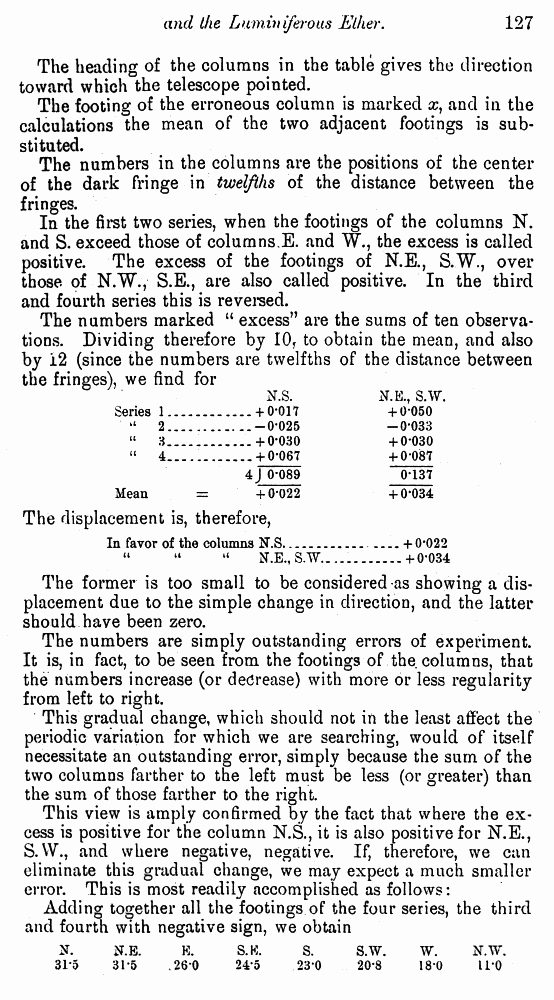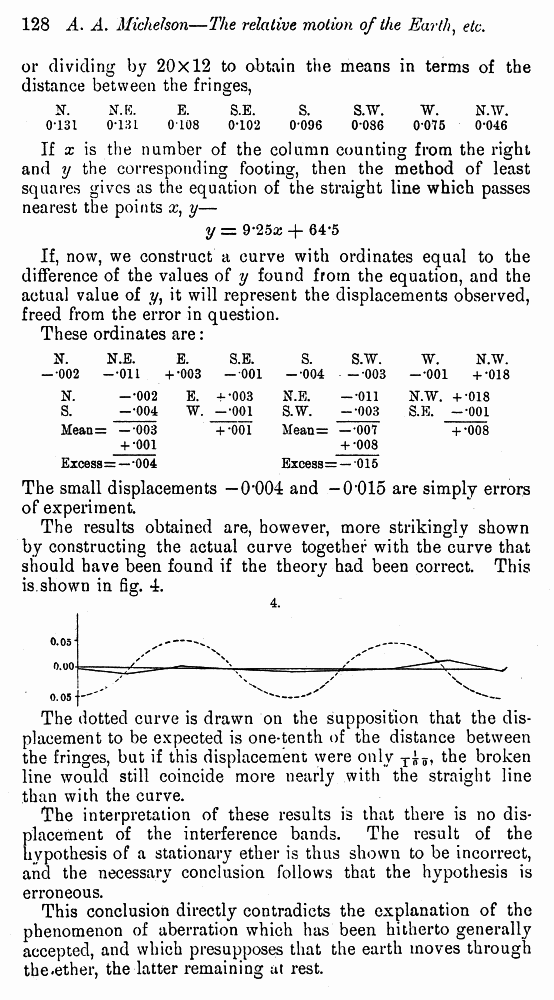# Albert A. Michelson. The Relative Motion of the Earth and the Luminiferous Ether // American Journal of Science, 1881, 22: Page 127

┬ ĒÓ„ÓļŅ   ─­¾ŃĶÕ ¶Ņ­ņÓ“¹   <<<     č“­ÓĒĶ÷Ó 8   >>>1  2  3  4  5  6  7  8 9  10

The heading of the columns in the table gives the direction toward which the telescope pointed.

The footing of the erroneous column is marked x) and in the calculations the mean of the two adjacent footings is substituted.

The numbers in the columns are the positions of the center of the dark fringe in twelfths of the distance between the fringes.

In the first two series, when the footings of the columns N. and S. exceed those of columns.E. and W., the excess is called positive. The excess of the footings of N.E., S.W., over tbose of N.W., S.E., are also called positive. In the third and fourth series this is reversed.

The numbers marked ō excessö are the sums of ten observations. Dividing therefore by I0f to obtain the mean, and also by 12 (since the numbers are twelfths of the distance between the frinees\ we find for

 N.S. N.E., S.W. Series 1......... + 0*017 + 0*050 ' ō 2......... -0*025 -0*033 ō 3_________ + 0*030 + 0*030 ō 4......... ____+ 0*067 + 0*087 4J 0*089 0*137 Mean = + 0*022 + 0*034

The displacement is, therefore,

In favor of the columns 1T.S_________________+ 0'022

ō ō ō N.B., S.'W............+0-034

The former is too small to be considered-as showing a displacement due to the simple change in direction, and the latter should have been zero.

The numbers are simply outstanding errors of experiment. It is, in fact, to be seen from the footings of the columns, that the numbers increase (or decrease) with more or less regularity from left to right.

This gradual change, which should not in the least affect the periodic variation for which we are searching, would of itself necessitate an outstanding error, simply because the sum of the two columns farther to the left must be less (or greater) than the sum of those farther to the right.

This view is amply confirmed by the fact that where the excess is positive for the column N.S., it is also positive for N.E., S.W.7 and where negative, negative. If, therefore, we can eliminate this gradual change, we may expect a much smaller error. This is most readily accomplished as follows:

Adding together all the footings of the four series, the third and fourth with negative sign, we obtain

 N. N.E. E. S.K. S. S.W. W. N.W. 31-5 31-5 .26-0 24-5 23-0 20*8 18*0 11*0

or dividing by 20x12 to obtain the means in terms of the distance between the fringes,

 N. N.E. E. S.E. S. S.W. W. N.W. -Ģ002 -ĢOil + Æ003 -001 -Ģ004 -Ģ003 -Ģ001 + Ģ018 N. -Ģ002 E. + Ģ003 N.E. -Ģ011 N.W. + Ģ018 S. -Ģ004 W. -Ģ001 S.W. -Ģ003 S.E. -Ģ001 Mean = : --003 + *001 Mean= -Ģ007 + Ģ008 + Ģ001 + Ģ008 Excess = -Ģ004 Excess= = -015

The small displacements Ś 0'004 and -0Æ015 are simply errors of experiment.

The results obtained are, however, more strikingly shown by constructing the actual curve together with the curve that should have been found if the theory had been correct. This is.shown in fig. 4.

The dotted curve is drawn on the supposition that the displacement to be expected is one*tenth of the distance between the fringes, but if this displacement were only T^, the broken line would still coincide more nearly with the straight line than with the curve.

The interpretation of these results is that there is no displacement of the interference bands. The result of the hypothesis of a stationary ether is thus shown to be incorrect, and the necessary conclusion follows that the hypothesis is erroneous.

This conclusion directly contradicts the explanation of the phenomenon of aberration which has been hitherto generally accepted, and which presupposes that the earth moves through the.ether, the latter remaining at rest.

 N. N.E. E. S.E. S. S.W. W. N.AV. 0-131 0-131 0-108 0-102 0*096 0*086 0*075 0-046

If x is the number of the column counting from the right and y the corresponding footing, then the method of least squares gives as the equation of the straight line which passes nearest the points x)

y = 9æ25x + 64*5

If, now, we construct a curve with ordinates equal to the difference of the values of y found from the equation, and the actual value of y, it will represent the displacements observed, freed from the error in question.

These ordinates are: